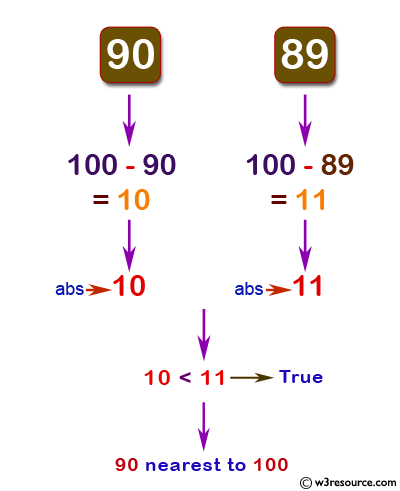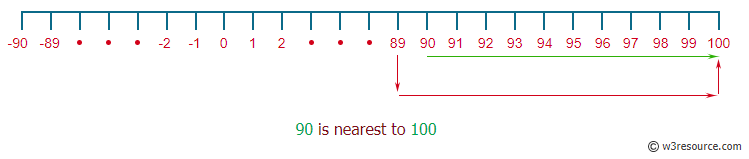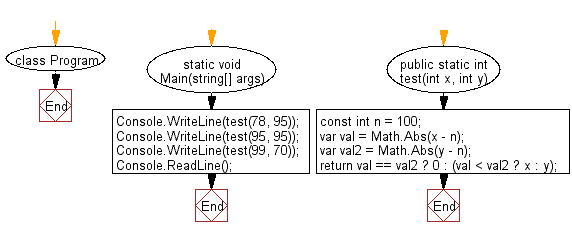﻿ C# - Find the nearest integer to 100 among two integers# C# Sharp Basic Algorithm Exercises: Check which number is nearest to the value 100 among two given integers

## C# Sharp Basic Algorithm: Exercise-19 with Solution

Write a C# Sharp program to check which number is nearest to the value 100 among two given integers. Return 0 if the two numbers are equal.

Pictorial Presentation 1:Pictorial Presentation 2:Sample Solution:

C# Sharp Code:

``````using System;
namespace exercises
{
class Program
{
static void Main(string[] args)
{
Console.WriteLine(test(78, 95));
Console.WriteLine(test(95, 95));
Console.WriteLine(test(99, 70));
}
public static int test(int x, int y)
{
const int n = 100;
var val = Math.Abs(x - n);
var val2 = Math.Abs(y - n);

return val == val2 ? 0 : (val < val2 ? x : y);
}
}
}
```
```

Sample Output:

```95
0
99```

Flowchart:C# Sharp Code Editor:

Improve this sample solution and post your code through Disqus

What is the difficulty level of this exercise?

Test your Programming skills with w3resource's quiz.

﻿